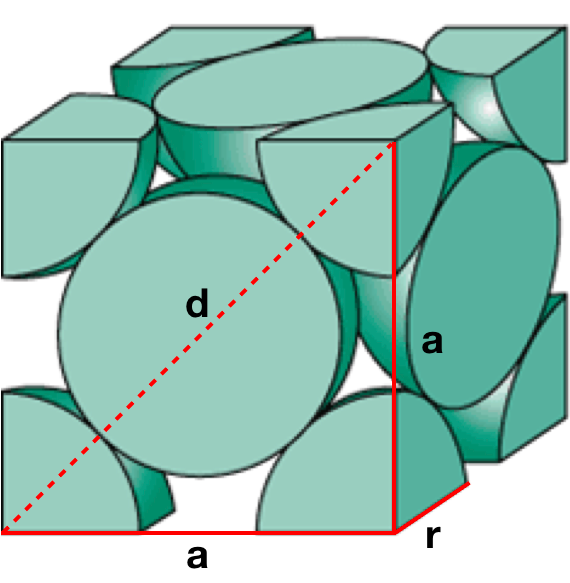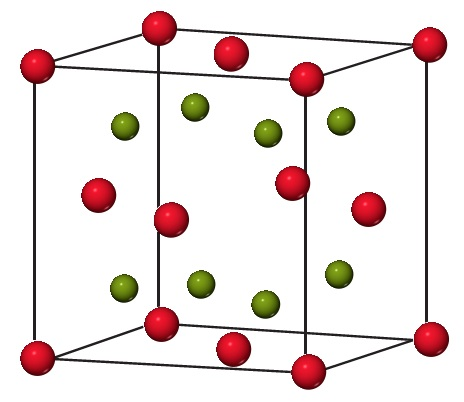# Problem: Sodium oxide (Na2O) adopts a cubic structure with Na atoms represented by green spheres and O atoms by red spheres.The unit cell edge length is 5.55 Å . Determine the density of Na2O.

###### FREE Expert Solution

We are being asked for the density of Na2O, given the unit cell edge length of 5.55 Å

Looking at the illustration, Na2O has face centered cubic structure.

Recall that the face-centered cubic lattice contains an atom in each of the faces of the cube and an atom in each of the corners

It looks like this:There are 4 atoms in a unit cell for a Face Centered Cubic structure (z=4).

88% (262 ratings)###### Problem Details
Sodium oxide (Na2O) adopts a cubic structure with Na atoms represented by green spheres and O atoms by red spheres.The unit cell edge length is 5.55 Å . Determine the density of Na2O.